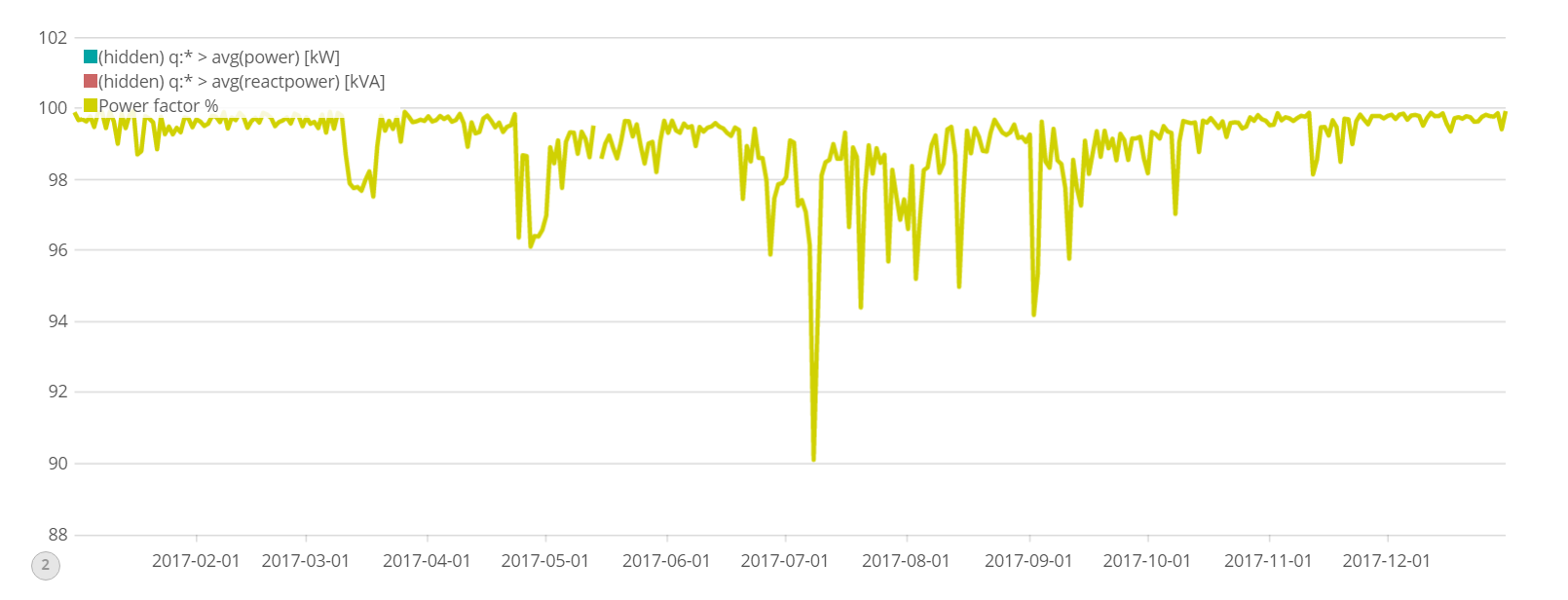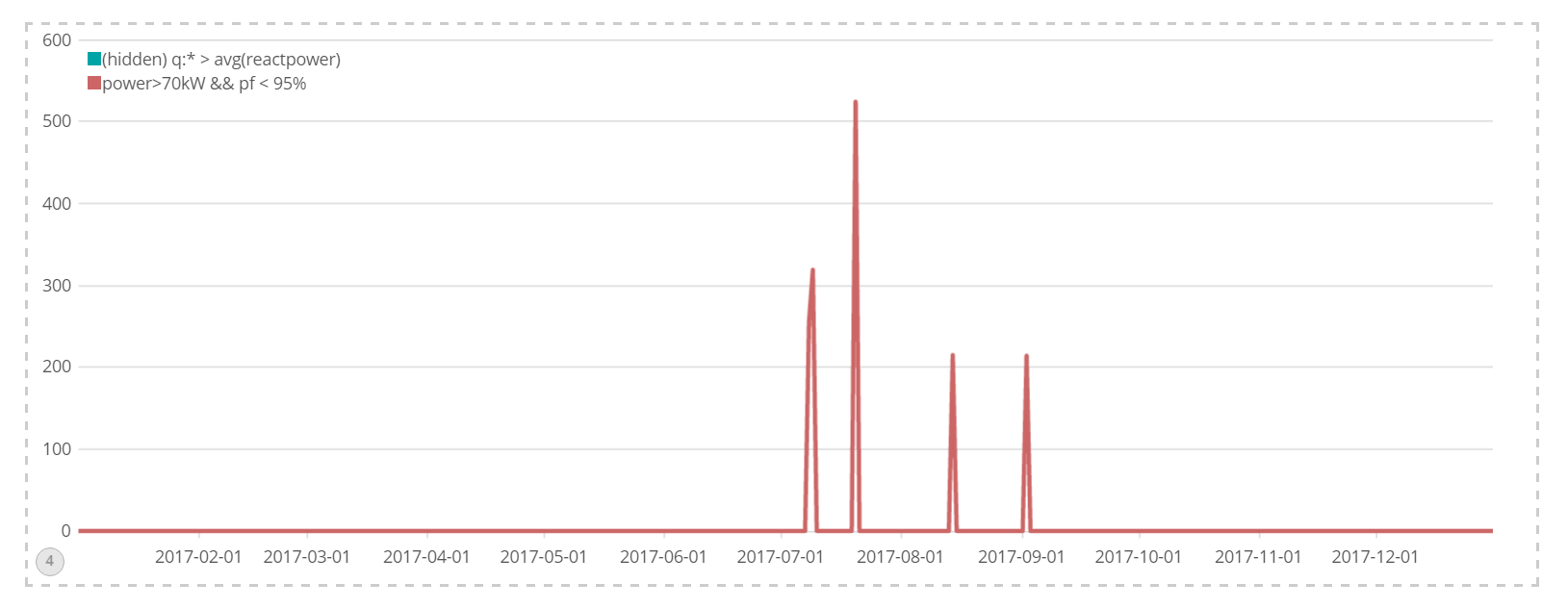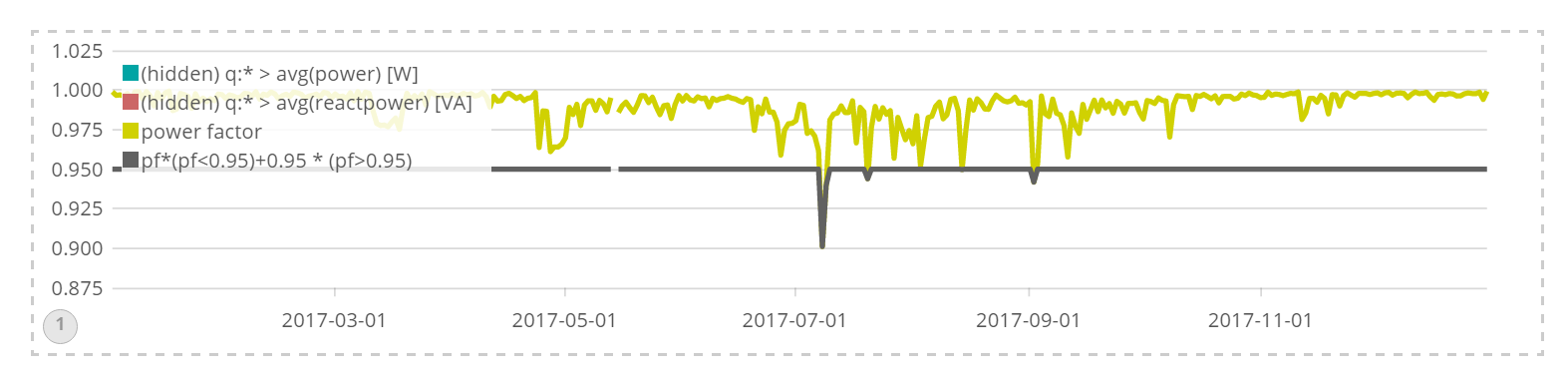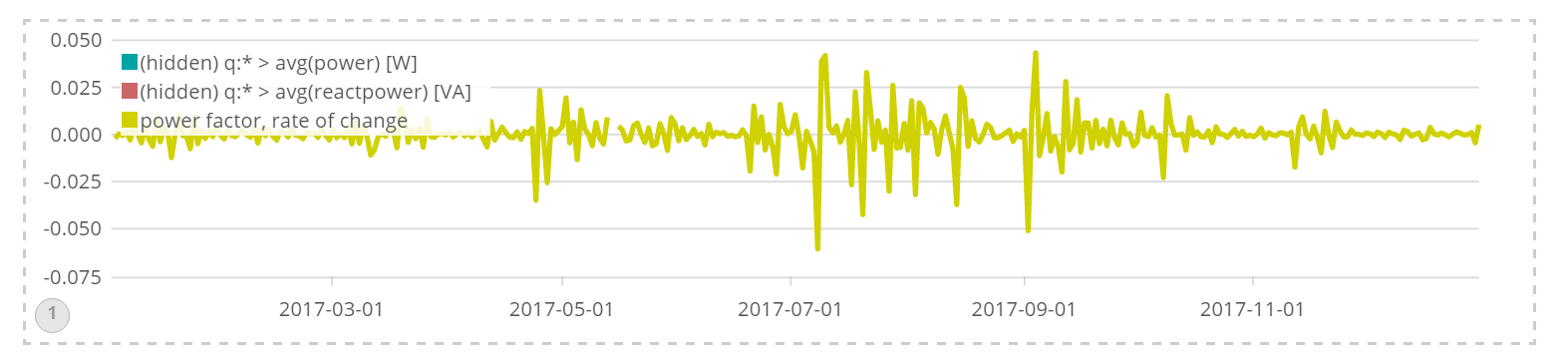User Stories

# Computation near the visualization layer

At Fermium LABS we use the Elastic Stack to monitor the behaviour of industrial machinery. While we can run various analytical softwares on top of it, there is really no tool like Kibana to dig into the data quickly.

Our mantra to keep the system light and usable is "analyze data only when needed, at the latest moment possible." The Elastic Stack fits nicely into this philosophy, keeping the cloud provider's bill down and allowing every person on our customer's team (and ours) to play on the same dataset stored in Elasticsearch.

We've been looking constantly to move more and more of the data analytics as close to the user and as far away from the database as possible.

The introduction of Timelion in Kibana 5.x allowed us to perform more flexible computations on top of the queries, such as derivatives, cumulative sums and applying filters.

### The problem

Engineers love math. They really do! We found that Timelion's native syntax was a bit distant from the algebraic equations automation engineers were used to.

Here's how you currently do (x+10)*1.1 in Timelion

.es(metric=x).add(10).multiply(1.1)

The expression would get further complicated if two values needed to be compared through some kind of mathematical formula, as often is the case when physics is involved.

### The solution

Fortunately, for every missing feature in Kibana there are endless expansion possibilities.

The goal was to allow the previous expression to be written neatly, with all the useful information between quotes, as follows:

.es(metric=x).math("(source + 10)* 1.1")

This syntax also allows all the math to be concentrated in a single equation and combine data from different Elasticsearch queries neatly.

Following this goal, we developed Mathlion, a small plugin to enable equation parsing and math in Timelion, based on the MathJS library.

It can do statistical operations, Boolean logic, deal with units of measurement and pretty much anything MathJS can do natively.

### Extending Timelion

Timelion's syntax is basically a chain of functions, the first of class datasource provides the list of values (seriesList), each subsequent of class chainable transforms those values.

In our case Mathlion's .math() function accept as a mandatory input the string (for example"source + 123") with the equation to parse provided by the user.

module.exports = new Chainable('math', {
args: [
{
name: 'inputSeries',
types: ['seriesList']
},
{
name: 'function',
types: ['string'],
help: 'The function to evaluate. Use \"source\" to refer to the preceding chainable. Check out mathlion.docs.fermiumlabs.com'
}
],
fn: function mathChain(args, tlConfig) {
//MAGIC HAPPENS HERE
return myOutputSeriesList;
}
});

The new functions needs to be wrapped in a NodeJS module and correctly exported in order to be installable as a plugin:

module.exports = function (kibana) {
return new kibana.Plugin({
name: 'mathlion',
require: ['timelion'],
init: function (server) {
}
});
};

Rashid Khan, original developer of Timelion and Kibana, maintains an example Timelion plugin on GitHub.

### The use case

In this example we have documents containing three numeric variables:

• reactpower (Reactive Power)
• power (Active Power)
• voltage (Voltage)

By itself, only "power" is somewhat useful. What we're really interested in is to calculate an indicator called "Power Factor" (pf) that needs to be kept above 95% to avoid penalties in the electric bill. The math is the following:Where |S| is the apparent power, P is the active power, Q the reactive power, pf% the reactive power in percentage.

Unfortunately, Timelion cannot natively do square roots. But we can with Mathlion.

.es(metric=avg:power)
.math("power=(source kW)")
.hide().label(null)

.es(metric=avg:reactpower)
.math("react=source kVA").hide()

.nop().math("(power / sqrt(react^2+power^2))*100")
.label("Power factor %")

The resulting graph:We can also use a Boolean expression to filter our data, for example to show only values where we are using a lot of power (more than 70kW) and also where the power factor is critically low pf<95

Boolean conditions can have a value of either 0 or 1. We can easily multiply their result to our incoming data to zero it out (if the condition is false) or pass it along (if true).

.es(metric=avg:reactpower)
.math("react=(source+0.000000001) ")
.hide()

.es(metric=avg:power)
.math("source * (source>70) * (( source/sqrt(react^2+source^2) ) < 0.95)")Since subsequent expressions can see the variables of the previous ones we can display graphs over graphs calculated on the same values.

.es(metric=avg:power)
.math("power=(source kW)")
.hide()

.es(metric=avg:reactpower)
.math("react=source kVA")
.hide()

//calculate the power factor; return the power factor
.nop()
.math("pf=power / sqrt(react^2+power^2);pf")

//re-plot over the power factor if <95
//else plot 95%
.nop()
.math("pf *(pf<0.95)+ 0.95 * (pf>0.95)")

The graph is redrawn in grey only when pf<95, otherwise it displays a horizontal line that indicates the threshold.All native Timelion functions can be chained to Mathlion .math(" ")function, for example to display the rate of change of the calculated power factor by appending .derivative()### Conclusions

Timelion is an incredible tool that allows an expressive syntax to be applied to Elasticsearch's queries outputs. For the edge cases and custom requirements that cannot be covered by Timelion itself it's easy to write plug-in functions in NodeJS.